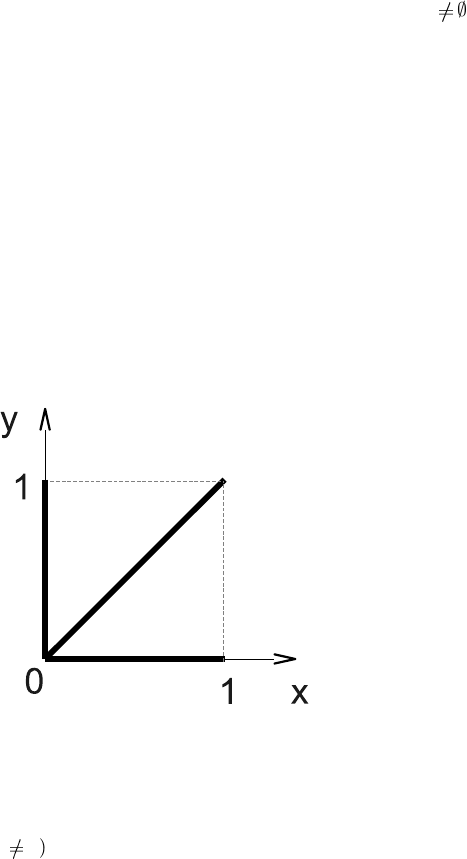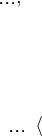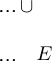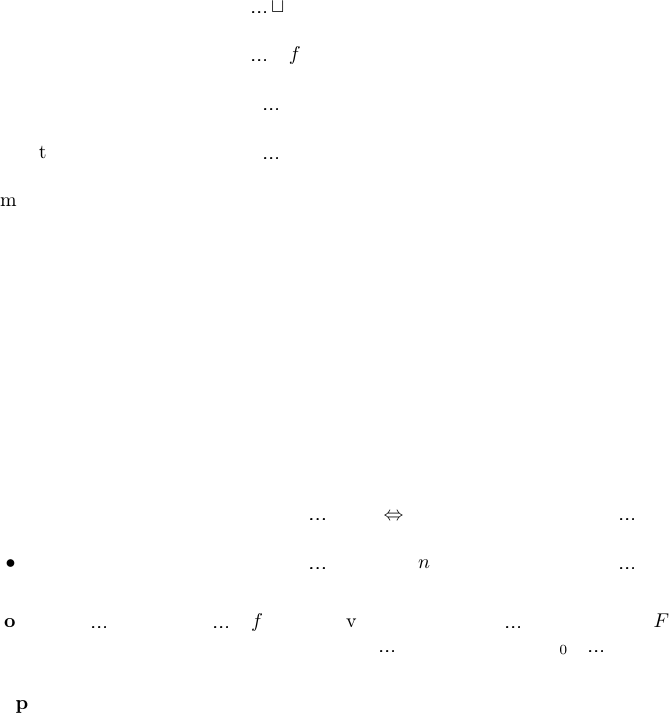Algebraic General Topology. Vol 1: Paperback / E-book || Axiomatic Theory of Formulas: Paperback / E-book

As an Amazon Associate I earn from qualifying purchases.

Total boundness of reloids
by Victor Porton
June 6, 2013
Abstract
I generalize total boundness of uniform spaces for arbitrary reloids (lters on a cartesian pro-
duct of sets). For reloids total boundness splits into two diﬀerent concepts: α-total boundness
and β-total boundness.
Notation
I call reloid a triple (A; B; F) where A, B are sets and F is a ﬁlter on the cartesian product A × B
of these sets. I will denote GR(A; B; F) = F for a reloid (A; B; F). Relo ids are a generalization
of uniform spaces. Source of a reloid is Src(A; B; F) = A and destination Dst(A; B; F) = B. I also
denote xyGR(A; B; F ) = {(A; B; f ) | f F }.
I will r efer to a pair f = (U; E) of a (small) set U and a binary relation E E × E as Rel-
endomorph ism. Furthermore Ob f = U and GR f = E.
I denote hE iX = {y | x X: x E y} for a binary relation E and set X and hE iX = hGR Ei X for
a Rel-endomorphism E.
I deﬁne composition of reloids (B; C; G) (A; B; F ) = (A; C; H) where H is the ﬁlter induced by
the ﬁlter base {g f | f F , g G}.
The reverse reloid is deﬁned by the formula (A; B; F )
1
= (B; A; F
1
).
I deﬁne partial order on the set of reloids as (A; B; F ) (A; B; G) F G. The set of reloids with
given source and destination is a complete lattice with join denoted and meet denotes .
See http://www.mathematics21.org/algebraic-general-topology.html for mor e de tails.
Thi ck binary relations
Deﬁnition 1. I will call α-thick and denote thick
α
(E) a Rel-endomorphism E when there exists
a ﬁnite cover S of Ob E such that A S: A × A GR E.
Deﬁnition 2. CS(S) =
S
{A × A | A S} for a collection S of sets.
Remark 3. CS means “cartesian squares”.
Obvious 4. A Rel-endomo rphism is α-thick iﬀ there exists a ﬁnite cove r S of Ob E such that
CS(S) GR E.
Deﬁnition 5. I will ca ll β-thick and de note thick
β
(E) a Rel -endomorphism E when iﬀ there
exists a ﬁnite set B such that hE iB = Ob E.
Proposition 6. thick
α
(E) thick
β
(E).
1Proof. Let thick
α
(E). Then there exists a nite cover S of the set Ob E such that A S:
A × A GR E. Without loss of generality assume A
for every A S. So A hE i{x
A
} for some
x
A
for every A S. So hE i{ x
A
| A S} =
S
{hE i{x
A
} | A S} = Ob E and thus E is β-thick.
Obvious 7. Let X be a set, A an d B are Rel-endorelations on X and B A. Then:
thick
α
(A) thick
α
(B);
thick
β
(A) thick
β
(B).
Example 8. There is a β-thick Rel-morphism which is not α-thick.
Proof. Consider the Rel-morphism on [0; 1] with the below graph:
Γ = {(x; x) | x [0; 1]} {(x; 0) | x [0; 1]} {(0; x) | x [0; 1]}.
Γ is β-chick because hΓi{0} = [0; 1].
To prove that Γ is not α-thick it’s enough to prove that every set A such that A × A Γ is ﬁnite.
Suppose for the contrary th at A is inﬁnite. Then A contains more than one non-zero points y, z
(y
z). Without loss of generality y < z. So we have that (y, z) is not of the form (y; y) nor (0;
y) nor (y; 0). Theref ore A × A isn’t a subset of Γ.
Totally bounded en do-reloids
The below is a straightforward generalization of the customary deﬁnition of to tally bounded sets
on uniform spaces (it’s proved be low that for uniform spaces the below deﬁnitions are equivalent).
Deﬁnition 9. An endoreloid f is α-totally bounded (totBound
α
(f )) if every E xyGR f is α-thick.
Deﬁnition 10. An endo reloid f is β -totally bounded (totBound
β
(f )) if every E xyGR f is β-
thick.
Remark 11. We could rewrite the above deﬁnitions in a more algebraic way like xyGR f thick
α
(with thick
α
would be deﬁned as a se t rather than as a predicate), but we don’t really need thi s
simpliﬁcation.
Proposition 12. If an endoreloid is α-totally bounded then it is β-totally bounded.
2Proof. Because thick
α
(E) thick
β
(E).
Proposition 13. If an endoreloid f is reﬂexive and Ob f is ﬁnite then f is both α-totally bounded
and β-totally bounded.
Proof. It enough to prove that f is α-totally bounded. Really, every E xyGR f is reﬂexive.
Thus {x} × {x} E for x Ob f and thus {{x} | x Ob f } is a sought for ﬁnite cover of Ob f.
Obvious 14.
A principal endo-reloid induced by a Rel-morphism E is α-totally bounded iﬀ E is α-thick.
A principal end o-reloid induced by a Rel-morphism E is β-totally bounded iﬀ E is β-thick.
Example 15. There is a β-totally bounded endoreloid which is not α-totally bounded.
Proof. It follows from the example above and properties of principal endoreloids.
Special case of uniform spaces
Deﬁnition 16. Uniform space is essentially the same as symmetric, reﬂexive and transitive endo-
reloid.
Exercise 1. Prove that it is essentially the same as the standard deﬁnition of a uniform space (see Wikipedia
or PlanetMath).
Theorem 17. Let f is such a endoreloid that f f
1
f . Then f is α-totally bounded iﬀ it is
β–totally bounded.
Proof.
. Proved above.
. For every ε GR f we have that hεi{c
0
},
, hεi{c
n
} covers the space. hεi{c
i
} × hεi{c
i
}
ε ε
1
because for x hεi{c
i
} (the same as c
i
hε
1
i{x}) we have hhεi{c
i
} × hεi{c
i
}i{x} =
hεi{c
i
} hεihε
1
i{x} = hε ε
1
i{x}. There exists ε
GR f such that ε ε
1
ε
because
f f
1
f. Thus for every ε
we have hεi{c
i
} × hεi{c
i
} ε
and so
hεi{c
0
},
, hεi{c
n
}.
is a sought for ﬁnite cover.
Corollary 18. A uniform space is α-totally bounded iﬀ it is β-totally bounded .
Proof. From the theorem and the deﬁ nition of uniform spaces.
Relationshi ps with other properties
Theorem 19. Let µ and ν are endoreloids. Let f is a principal C
(µ; ν) continuous, monovalued,
surjective re loid. Then if µ is β-totally bounded then ν is also β-totally bounded.
3Proof. Let ϕ is the monovalued, surjective f unction, which induce s the relo id f .
We have µ f
1
ν f .
Let F GR ν. Then there exists E GR µ such that E ϕ
1
F ϕ.
Since µ is β-totally bounded, ther e exists a ﬁnite subset A of Ob µ such that hEiA = Ob µ.
We claim hF ihϕiA = Ob ν.
Indeed let y Ob ν be an arbitrary point. Since ϕ is surjective, there exists x Ob µ such that
ϕx = y. Since hE iA = Ob µ there exists a A such that a E x and thus a (ϕ
1
F ϕ) x. So
(ϕa; y) = (ϕa; ϕx) F . Therefore y hF ihϕiA.
Theorem 20. Let µ and ν are endoreloids. Let f is a principal C
′′
(µ; ν) continuous, surjective
reloid. Then if µ is α-totally bounded then ν is also α-totally bounded.
Proof. Let ϕ is the s urjective bina ry re lat ion which induces the re loid f.
We have f µ f
1
ν.
Let F GR ν. Then there exists E GR µ such that ϕ E ϕ
1
F .
There exists a ﬁnite cover S of Ob µ such that
[
{A × A | A S} E.
Thus ϕ (
S
{A × A | A S }) ϕ
1
F that is
S
{hϕiA × hϕiA | A S} F .
It remains to prove that {hϕiA | A S } is a cover of Ob ν. It is true because ϕ is a surjection and
S is a cover of Ob µ.
A stronger statement (principality req uirement removed):
Conjecture 21. The image of a uniformly continuous entirely deﬁned monovalued s urjective reloid
from a (α-, β-)totally bounded endoreloid is also (α-, β-)totally bounded.
Can we remove the requirement to be entirely deﬁned from the above conjecture?
Question 22. Under which conditions it’s true that join of (α-, β-) totally bo unded reloids is also
totally bounded?
We may consider also the following predicates expressing diﬀerent kinds of what is intuiti vely is
understood as boundness. Their usefulne ss is unclear, but I present them for completeness.
E GR f n N: thick
α
(E
n
)
E GR f n N: thick
β
(E
n
)
E GR f n N: thick
α
(E
0
E
n
)
E GR f n N: thick
β
(E
0
E
n
)
n N: totBound
α
(f
n
)
4n N: totBound
β
(f
n
)
n N: totBound
α
(f
0
f
n
)
n N: totBound
β
(f
0
f
n
)
totBound
α
(f
0
f
1
f
2
)
totBound
β
(f
0
f
1
f
2
)
Some of the above deﬁned predicates are equivalent:
Proposition 23.
E GR f n N: thick
α
(E
n
) n N: totBound
α
(f
n
).
E GR f n N: thick
β
(E
n
) n N: totBound
β
(f
n
).
Proof. Because every F GR f
n
is a superset of E
n
for some E GR f.
Proposition 24.
E GR f n N: thick
α
(E
0
E
n
) n N: totBound
α
(f
0
f
n
).
E GR f n N: thick
β
(E
0
E
n
) n N: totBound
β
(f
0
f
n
).
Proof. f
0
f
n
= f
0
f
n
. Thus every F GR(f
0
f
n
) we have F f
k
, thus F E
k
k
for all k for some E
k
GR f and so F E
0
E
n
where E = E
0
E
k
GR f .
Proposition 25. All predicates in th e above list are pairwise equivalent in the case if f is a
uniform space .
Proof. Because f f = f.
5